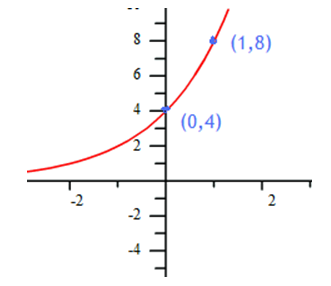Modeling A Graph

In order to write the function that models an exponential graph, find at least two points on the graph. If the y-intercept (0, a) is easily determined, select it as one of the two points. Otherwise, select two points with integer coordinates to make the computation easier. Then calculate the a and b-values.

Example Write a function to model the graph.Step 1. Determine the y-intercept.

Notice (0, 4) is on the graph, therefore a = 4 and y = 4 . bx

Step 2. Determine another point on the graph and substitute it into x and y in the equation, then solve for b.

Notice (1, 8) is on the graph, use it in the equation.

8 = 4 . b1

2 = b

Therefore, y = 4 . 2x models this graph.